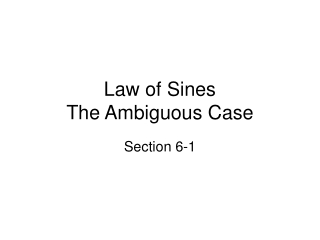DownloadDownload PresentationLaw of Sines The Ambiguous Case

# Law of Sines The Ambiguous Case

Télécharger la présentation## Law of Sines The Ambiguous Case

- - - - - - - - - - - - - - - - - - - - - - - - - - - E N D - - - - - - - - - - - - - - - - - - - - - - - - - - -
##### Presentation Transcript

1. Law of Sines The Ambiguous Case Section 6-1

2. In geometry, triangles can be uniquely defined when particular combinations of sides and angles are specified … this means we can solve them! Angle Side Angle - ASA Angle Angle Side – AAS We solved these using Law of Sines Then there are these theorems … Side Side Side – SSS Side Angle Side – SAS We’ll soon solve these using the Law of Cosines (section 6.2)

3. There’s one left … Side Side Angle - SSA There’s a problem with solving triangles given SSA … You could find… • No solution • One solution • Two solutions In other words … its AMBIGUOUS … unclear Let’s take a look at each of these possibilities.

4. Remember now … the information we’re given is two consecutive sides and the next angle … • If this side isn’t long enough, then we can’t create a triangle … no solution So, then, what is the “right” length so we can make a triangle? An altitude … 90 degree angle … a RIGHT triangle! Turns out this is an important calculation … it’s a = b sin θ • If a = b sin θ, then there is only one solution for this triangle. • The missing angle is the complement to θ • The missing side can be found using Pythagorean theorem of trigonometry. b a θ

5. a What if side a is a little too long … what would that look like? a > b sin θ This leg can then either swing left … or right. So? … which one of these triangles do you solve? … BOTH! • First, solve the acute triangle … and find angle B by Law of Sines! • Then solve for the remaining parts of the acute triangle b θ B

6. a Lastly, solve the obtuse triangle … This next step is critical … angle B’ is ALWAYS the supplement to angle B. B’ = 180 – m< B • Next, solve the remaining parts of the obtuse triangle. b θ B’ B

7. a Here’s the last scenario while θ is acute … What if side a is larger than side b? • In this case, only one triangle can exist … an acute triangle which can easily be solved using law of sines. Too long to create a triangle on this side. θ